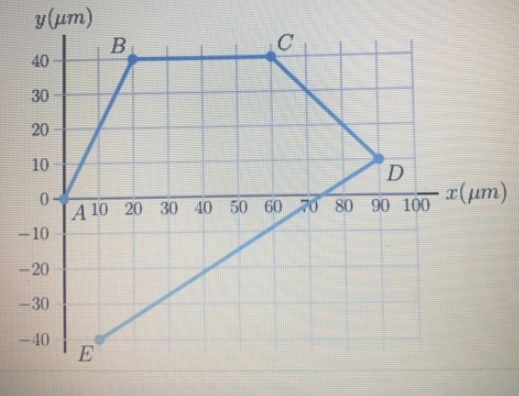# Problem: The bacterium E coli is a single-celled organism that lives in the gut of healthy human and animals. When grown in a uniform medium rich in salts and amino acids, these bacteria swim along zig-zag paths at a constant speed of 11 micometers per second. The figure shows the trajectory of an E. coli as it moves from the origin at point A to Point E.a. Calculate the total distance traveled. b. Calculate the magnitude of the net displacement for the entire motion. c. What's is the bacteriums average speed for the entire trip? d. What is the magnitude of the bacteriums average velocity for the entire trip?

###### FREE Expert Solution

We're going to use the equation for vector magnitude:

$\overline{)\mathbf{|}\stackrel{\mathbf{⇀}}{\mathbit{A}}\mathbf{|}{\mathbf{=}}\sqrt{{{\mathbit{A}}_{\mathbit{x}}}^{\mathbf{2}}\mathbf{+}{{\mathbit{A}}_{\mathbit{y}}}^{\mathbf{2}}}}$

a.

The total distance traveled is given by:

d = AB + BC + CD + DE

93% (357 ratings)###### Problem Details

The bacterium E coli is a single-celled organism that lives in the gut of healthy human and animals. When grown in a uniform medium rich in salts and amino acids, these bacteria swim along zig-zag paths at a constant speed of 11 micometers per second. The figure shows the trajectory of an E. coli as it moves from the origin at point A to Point E.

a. Calculate the total distance traveled.

b. Calculate the magnitude of the net displacement for the entire motion.

c. What's is the bacteriums average speed for the entire trip?

d. What is the magnitude of the bacteriums average velocity for the entire trip?Frequently Asked Questions

What scientific concept do you need to know in order to solve this problem?

Our tutors have indicated that to solve this problem you will need to apply the Intro to Motion in 2D: Position & Displacement concept. You can view video lessons to learn Intro to Motion in 2D: Position & Displacement. Or if you need more Intro to Motion in 2D: Position & Displacement practice, you can also practice Intro to Motion in 2D: Position & Displacement practice problems.

What professor is this problem relevant for?

Based on our data, we think this problem is relevant for Professor Tsai's class at UCSD.